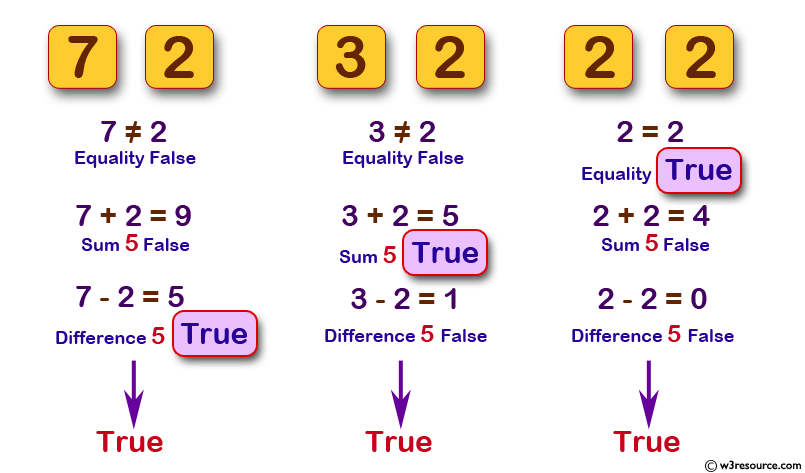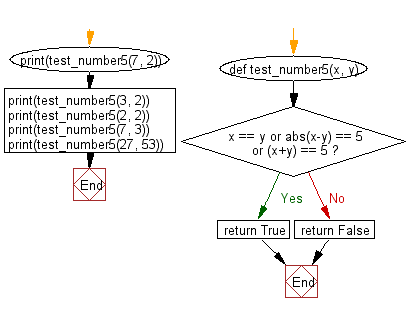﻿ Python: Return true if the two given integer values are equal or their sum or difference is 5 - w3resource# Python: Return true if the two given integer values are equal or their sum or difference is 5

## Python Basic: Exercise-35 with Solution

Write a Python program which will return true if the two given integer values are equal or their sum or difference is 5.

Pictorial Presentation:Sample Solution:

Python Code:

``````def test_number5(x, y):
if x == y or abs(x-y) == 5 or (x+y) == 5:
return True
else:
return False
print(test_number5(7, 2))
print(test_number5(3, 2))
print(test_number5(2, 2))
print(test_number5(7, 3))
print(test_number5(27, 53))
```
```

Sample Output:

```True
True
True
False
False
```

Flowchart:## Visualize Python code execution:

The following tool visualize what the computer is doing step-by-step as it executes the said program:

Python Code Editor:

Have another way to solve this solution? Contribute your code (and comments) through Disqus.

What is the difficulty level of this exercise?

Test your Python skills with w3resource's quiz

﻿

## Python: Tips of the Day

Try-catch-else construct:

```try:
foo()
except Exception:
print("Exception occured")
else:
print("Exception didnt occur")
finally:
print("Always gets here")
```